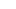# Paper Plate Math Facts

Need a fresh new way to practice math facts?  Instead of worksheets, pull out the paper plates and clothespins!  This simple, hands-on math activity is perfect for your Kindergarten, 1st or 2nd grade child.My kindergartner has recently been working on learning her math facts up to 5.
To focus on addition facts +2, I put together this simple math activity that doubles as a busy bag.
This is great math practice for Kindergarten, 1st grade, and 2nd grade to gain fluency when adding numbers.

# Paper Plate Math Facts

Use it when you need a few minutes to prepare lunch, while working with another kiddo, or while you are traveling in the car.  This Paper Plate Math Facts is super versatile and easy to put together.Supplies:
• paper plate
• clothespin
• markers

You could write out a whole set of math facts on several paper plates, but we chose to work on +2.

In the middle of the paper plate, I wrote +2 and then around the paper plate I wrote the numbers 0-5.

Next, I prepped the clothespins by writing the sum of each math fact at the end of the clothespin.

With the paper plate prepped, you child will now be able to work on their math facts!

Your child will add 2 to each of the numbers around the paper pate: 2+2, 5+2, 1+2, 3+2, 0+2, 4+2 then clip the correct clothespin sum at that fact.Thus 2+2 is 4, so the clothespin with the number four on it will be clipped by the number 2.  4+2 is six, so the clothespin with the number 6 on the end will be clipped at the number 4.

Have your child continue clipping the sums around the paper plate until they have completed each math fact.

For an element of self correction, I wrote each sum in a different color marker and then drew a small circle o the back of the paper plate.Enjoy this simple, fun way to practice math facts!  Here’s more hands-on ways to practice math facts!